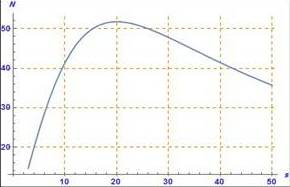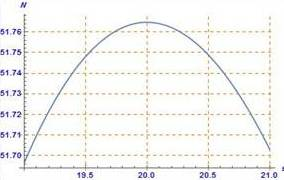# the greatest numbers of cars travel the highway safely### Precalculus: Mathematics for Calcu...

6th Edition
Stewart + 5 others
Publisher: Cengage Learning
ISBN: 9780840068071### Precalculus: Mathematics for Calcu...

6th Edition
Stewart + 5 others
Publisher: Cengage Learning
ISBN: 9780840068071

#### Solutions

Chapter 2.3, Problem 53E
To determine

## To find : the greatest numbers of cars travel the highway safely

Expert Solution

### Explanation of Solution

Given information : The question is

A highway engineer wants to estimate the maximum number of cars that can safely travel a particular highway at a given speed. Each car is 17ft. long and travels at a speed s

N(s)=88s17+17(s20)2

Graph : The graph of N as a function of s is shown

There appears to be a maximum between s=15&s=20

Using the maximum command,

The maximum value of N is about 51.7647 and it occurs when s=20Interpretation : The greatest number of cars travel the highway is at s=20 because the maximum value of N is about 51.7647

### Have a homework question?

Subscribe to bartleby learn! Ask subject matter experts 30 homework questions each month. Plus, you’ll have access to millions of step-by-step textbook answers!